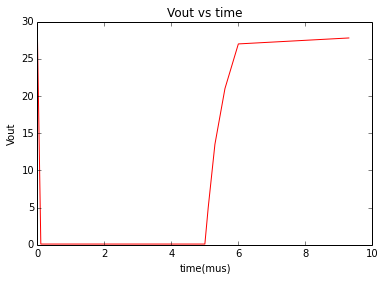# Chapter 6: Power Switches¶

### Example 6.1,Page 274¶

In :
#finding Ic,Pload,Pq,Ibase,Rbase

#initialisation of variable
from math import pi,tan,sqrt,sin,cos,acos,atan
Vs=28.0;#V
Vi=5.0;#V
Rl=15.0;#ohm
Vc=.8;#V
b=20.0;

#calculation
Ic=(Vs-Vc)/Rl;
Pl=Ic**2*Rl;
Pq=Ic*Vc;
Ib=Ic/b*1000;
Rb=(Vi-.6)/Ib;

#result
print "collector current is",round(Ic,2), "A"
print "transistor power is",round(Pq,2), "W"
print "least value of base current is",round(Ib,2), "mA"
print "max value of base resistance is",round(Rb*100,2), "ohm"
print ('thus pick Rb=33ohm')

collector current is 1.81 A
transistor power is 1.45 W
least value of base current is 90.67 mA
max value of base resistance is 4.85 ohm
thus pick Rb=33ohm


### Example 6.4,Page 282¶

In :
#finding Pload,Pq,Iload,resistances

#initialisation of variable
from math import pi,tan,sqrt,sin,cos,acos,atan
Vd=28.0;#V
f=100.0;#frequency
I=50.0;#current
Rl1=560.0;
Vp=2.4;
Ib=500.0;#microAmp
D=.5;#duty cycle

#calculation
Rl=(Vd-.3)/I;
Rb=(Vp-.9)/Ib;
Vl=Vd-.3;
Ip=Vl/Rl1;
Pl=D*Vl*Ip;
Pq=D*Ip*.3;

#result
print('thus pick Rl=560ohm')
print "max value of Rb is",round(Rb*1000,2),"kohm"
print('thus pick Rb=2.2kohm')
print "power delivered is",round(Pq*1000,2), "mW"

load resistance is 554.0 ohm
thus pick Rl=560ohm
max value of Rb is 3.0 kohm
thus pick Rb=2.2kohm
power delivered is 7.42 mW


### Example 6.5,Page 286¶

In :
#finding time of rise

#initialisation of variable
from math import pi,tan,sqrt,sin,cos,acos,atan
C=640.0;#capacitor
R2=3.3;#kohm

#calculation
t1=2.2*R1*C;
t2=2.2*R2*C;

#result
print "time of rise is",round(t1/1000,2), "ns"
print "time of rise is",round(t2/1000,2), "microsec"

time of rise is 788.48 ns
time of rise is 4.65 microsec


### Example 6.6,Page 287¶

In :
#finding Rpick up,time of rise

#initialisation of variable
%matplotlib inline
import numpy as np
import matplotlib.pyplot as plt
Vol=0.7;
Iol=40.0/1000;#current
Rpullup1=680.0;
C=640.0;
Epullup=28.0;
#for plotting
x=[0, .1, 1.9, 4.1, 5, 5.1, 5.3, 5.6, 6.0, 9.3];
y=[27.8, .1, .1, .1, .1, 5, 13.5, 21.0, 27.0, 27.8];

#calculation
Rpullup=(Epullup-Vol)/Iol;
trise=2.2*Rpullup1*C;
plt.plot(x,y,'r');
plt.xlabel ('time(mus)')
plt.ylabel ('Vout')
plt.title ('Vout vs time')

#result
print "resistance is",round(Rpullup,2), "ohm"
print('pick up resistance=680 ohm');
print "rise time is",round(trise/1000,2), "ns"

resistance is 682.5 ohm
pick up resistance=680 ohm
rise time is 957.44 ns### Example 6.7,Page 289¶

In :
#finding worst case resistance and power characteristics

#initialisation of variable
from math import pi,tan,sqrt,sin,cos,acos,atan
R1=.11;#resistance
Vd=28.0;#voltage
R2=6.0;#ohm
D=.4;#duty cycle
Q=62.0;

#calculation
Ro=2.6*R1;
Ip=Vd/(R2+Ro);
Vl=Ip*R2;
Pl=D*Vl*Ip;
Vq=Ip*Ro;
Pq=D*Vq*Ip;
T=40+round(Pq*10)/10*Q;

#result
print "worst case resistance is",round(Ro,3), "ohm"
print "drop voltage is",round(Vq,2), "V"
print "power is",round(Pq*10,2)/10, "W"
print "temperature is",round(T,2), "deg.C"

worst case resistance is 0.286 ohm
drop voltage is 1.27 V
power is 2.27 W
temperature is 182.6 deg.C


### Example 6.8,Page 292¶

In :
#finding voltage

#initialisation of variable
from math import pi,tan,sqrt,sin,cos,acos,atan
L=10.0;#inductor
I=4.5;#current
t=300.0#time

#calculation
V=L*I/t;

#result
print "voltage is",round(V*1000,2), "V"

voltage is 150.0 V


### Example 6.9,Page 298¶

In :
#finding resistance and power characteristics

#initialisation of variable
from math import pi,tan,sqrt,sin,cos,acos,atan
V1=.8;#voltage
V2=2.4;#voltage
D=.8;#duty cycle
Tj=150.0;#degreeC
Ta=40.0;#degreeC
Vd=28.0;
Vo=.7;
I=40.0;#mA;
R1=330;
R2=360;
Vn1=28;
Vn2=.7;

#calculation
k=(Vd-Vo)/I;
Vg=R2*Vd/(R1+R2)+Vn2;
Vgs=Vg-Vd;
Vl=Vd*Rl/(Rl+.57);
Il=Vl/Rl;
Pl=D*Vl*Il;
Vq=Il*.57;
Pq=D*Vq*Il;
Q=(Tj-Ta)/Pq-3.7;

#result
print "R1+R2 is",round(k*1000,2), "ohm"
print('pick R1=330ohm & R2=360ohm as they divide Vd setting 8V<Vg<18V')
print "node voltage for V1 is",round(Vn1,2),"V"
print "node voltage for V2 is",round(Vn2,2), "V"
print "gate voltage is",round(Vg,2), "V"
print "gate & source diff is",round(Vgs,2), "V"
print "Pq is",round(Pq,2), "W"
print "thermal resistance is",round(Q,2), "degreeC/W"

R1+R2 is 682.5 ohm
pick R1=330ohm & R2=360ohm as they divide Vd setting 8V<Vg<18V
node voltage for V1 is 28.0 V
node voltage for V2 is 0.7 V
gate voltage is 15.31 V
gate & source diff is -12.69 V
Pq is 2.26 W
thermal resistance is 44.92 degreeC/W


### Example 6.10,Page 305¶

In :
#finding time

#initialisation of variable
from math import pi,tan,sqrt,sin,cos,acos,atan
I=40.0;#current
Q=44.0;#nC

#calculation
t=Q/I;

#result
print "time is",round(t,2), "micro s"

time is 1.1 micro s


### Example 6.11,Page 313¶

In :
#finding different voltages and power

#initialisation of variable
from math import pi,tan,sqrt,sin,cos,acos,atan
D=.85;#duty cycle
Ts=60.0;#degreeC
Vd=28.0;#voltage
R1=.375;
R2=.2;

#calculation
I=Vd/(R1+R2+Rl);
Vl1=I*Rl;
P=D*Vl*I;
Vh=I*R1;
Ph=D*Vh*I;
Vl=I*R2;
Pl=D*Vl*I;
Pic=Ph+Pl;
Pic=1.56;
Tj=150;
Ta=60;
Q=(Tj-Ta)/Pic-2.2;

#result
print "current is",round(I,2), "A"
print "power is",round(P,2), "W"
print "high side voltage is",round(Vh,2), "V"
print "high side power is",round(Ph,2), "W"
print "low side voltage is",round(Vl,2), "V"
print "low side power is",round(Pl,2), "W"
print "IC power is",round(Pic,2), "W"
print "thermal resistance is",round(Q,2), "degreeC/W"

current is 1.8 A